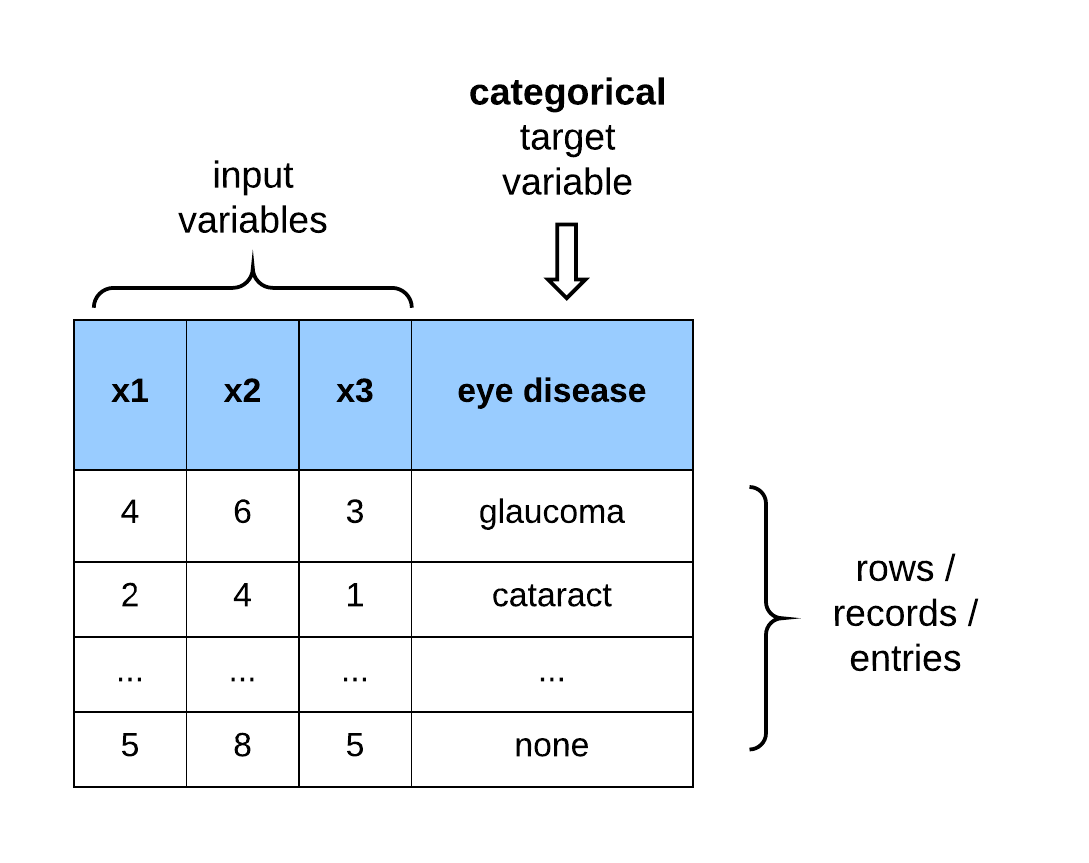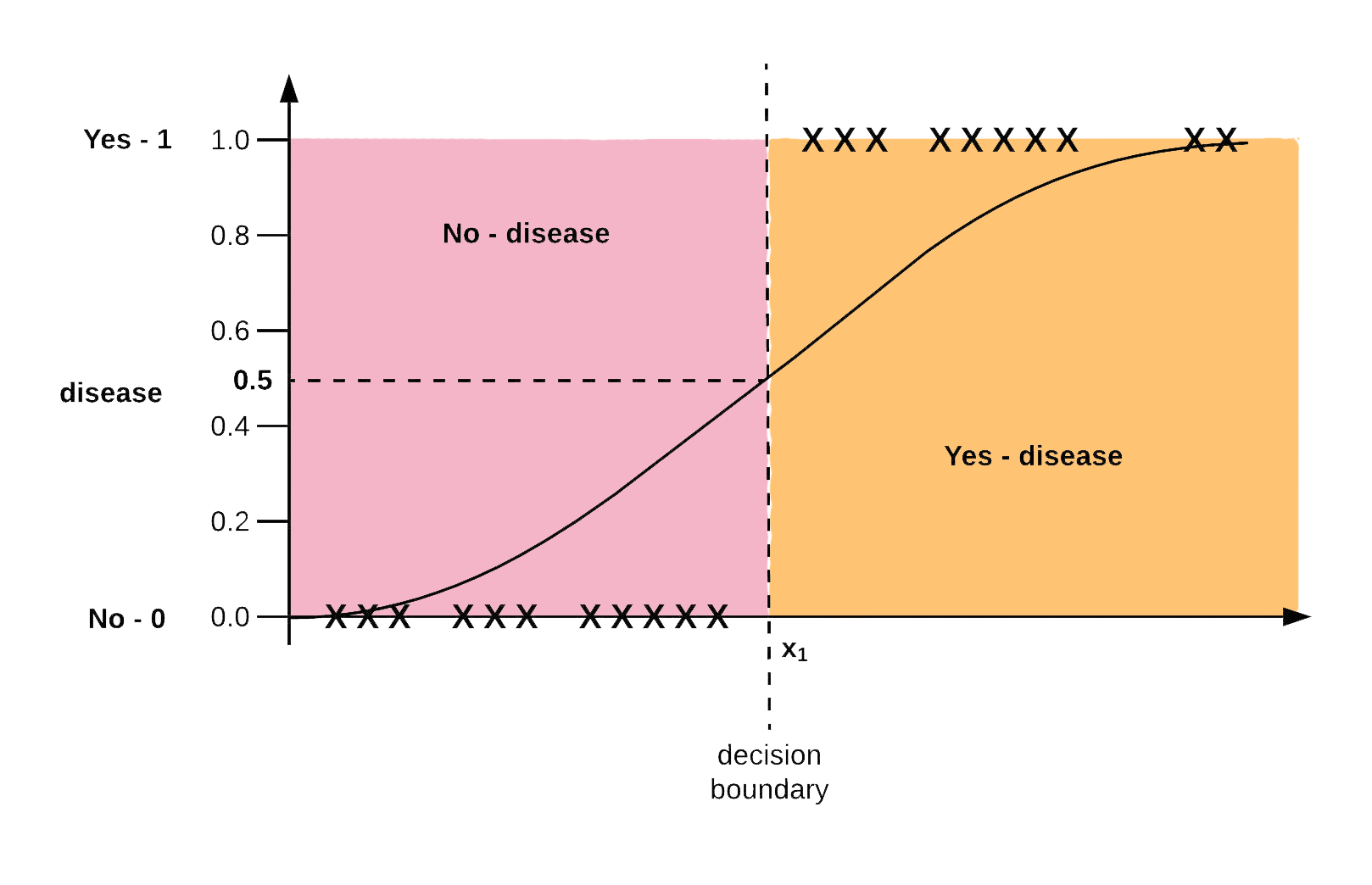Logistic regression is a supervised machine learning algorithm developed for learning classification problems. Remember that a classification learning problem is when the target variable (or the labels, or output) to be learned are categorical (i.e., they are qualitative). Interestingly, a good majority of machine learning problems are naturally framed as classification problems. The goal of logistic regression is to map a function from the input variables of a dataset to the output labels to predict the probability that a new example belongs to one of the output classes. The image below is an example of a dataset with categorical outputs.Figure 1: Dataset with categorical outputs

### The Logit or Sigmoid Model

The logistic function, also known as the logit or the sigmoid function is responsible for constraining the output of the cost function so that it becomes a probability output between 0 and 1. The sigmoid function is formally written as:

The logistic regression model is formally similar to the linear regression model. Only that it is acted upon by the sigmoid model. Below is the formal represemtation.

where, $0\leq h(t)\leq1$. The sigmoid function is graphically shown in the figure below:Figure 2: Logistic function

The sigmoid function, which looks like an $S$ curve, rises from 0 and plateaus at 1. From the sigmoid function shown above, as $X_1$ increases to infinity the sigmoid output gets closer to 1, and as $X_1$ decreases towards negative infinity, the sigmoid function outputs 0.

### The Logistic Regression Cost Function

The logistic regression cost function is formally written as:

The cost function also known as log-loss, is set up in this form to output the penalty made on the algorithm if $h(t)$ predicts one class, and the actual output is another.

### Multi-class classification/ multinomial logistic regression

In multi-class or multinomial logistic regression, the labels of the dataset contain more than 2 classes. The multinomial logistic regression setup (i.e., cost function and optimization procedure) is structurally similar to logistic regression, the only difference being that the output of logistic regression is 2 classes, while multinomial have greater than 2 classes.

At this point, we’ll introduce a critical function in machine learning called the softmax function. The softmax function is used to compute the probability that an instance belongs to one of K classes when $K > 2$. We will see the softmax function show up again when we discuss (artificial) neural networks.

The cost function for learning the class labels in a multinomial logistic regression model is called the cross-entropy cost function. Gradient descent is used to find the optimal values of the parameters $\theta$ that will minimize the cost function to predict the class with the highest probability estimate accurately.Figure 3: An illustration of multinomial regression